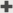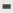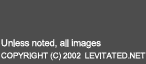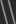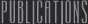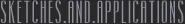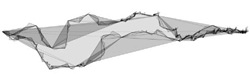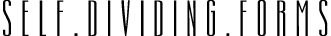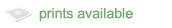The animated sketch above uses three pairs of subdividing lines to continually generate unique forms. There is no user interaction.

Intricate organic forms can be generated using recursively subdividing lines. By randomly offsetting the midpoint of each subdivision, an irregular overlapping line construct is produced.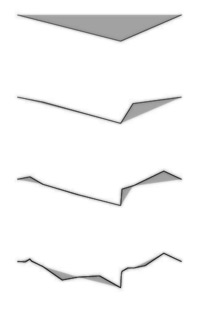figure each stage of the subdividing process calculates a randomly offset midpoint and repeats the process with the new segmentsThe key to the construction process is the definition of the subdividing line. In this example, the line is initially defined by two endpoints, a color, and a depth. The depth is used as a indicator of complexity. Because the subdividing line instantiates other subdividing lines, a measure of depth is required to keep the construct from becoming too complex. The line creates one object of its own, the midpoint.

The subdividing process is fairly simple. For any given line, a midpoint is calculated and randomly offset. Two new lines are then created using the original endpoints and the new midpoint. Each new line is assigned a depth value, indicating the number of times it has been divided. Once a particular limiting depth has been reached, the line stops dividing. The form is then complete.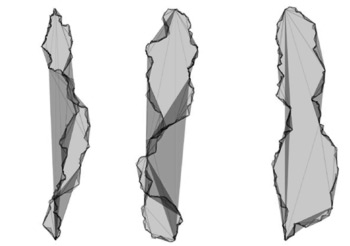figure three distinct recursively subdivided forms

The graphic composition of these self dividing forms is controlled using an increasingly opaque line, a nearly transparent fill, and a color transformation revolving around a fixed blue component.

Since the subdividing line is merely an extension of the line, there are many possible generative expansions. The pixie like figures seen in the image below were generated using only three subdividing lines each. Their sweeping movements were created by modifing the drawn line into a curve approximating the direction of subdivision.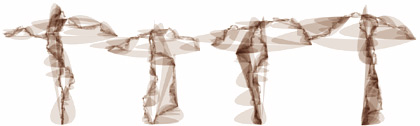figure dancing pixies composed initially of only twelve subdividing lines

This project was inspired by the countless implementations of subdivision in fractal landform generation.

jtarbell, September 2002